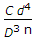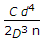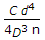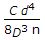# Mechanical Engineering - Strength of Materials

Exercise : Strength of Materials - Section 8
1.
A pressure vessel is said to be a thin shell when the ratio of wall thickness of the vessel to its diameter is __________ 1/10.
equal to
less than
greater than
Explanation:
No answer description is available. Let's discuss.

2.
According to Euler's column theory, the crippling load of a column is given by p = π2 EI/Cl2. In the Euler's formula, the value of C for a column with one end fixed and the other end hinged, is 1/2.
True
False
Explanation:
No answer description is available. Let's discuss.

3.
A tensile test is performed on a mild steel round bar. Its diameter after fracture will
remain same
increase
decrease
Explanation:
No answer description is available. Let's discuss.

4.
Two closely coiled helical springs 'A' and 'B' are equal in all respects but the diameter of wire of spring 'A' is double that of spring 'B' The stiffness of spring 'B' will be __________ that of spring 'A'
one-sixteenth
one-eighth
one-fourth
one-half